# PSAT Math : How to find the equation of a line

## Example Questions

← Previous 1

### Example Question #1 : How To Find The Equation Of A Line

Solve the equation for x and y.

x – 4y = 245

5x + 2y = 150

= 545/9

y = –1375/18

x = 3

y = 7

= 234/5

= 1245/15

= –1375/9

= 545/18

= 545/9

y = –1375/18

Explanation:

While solving the problem requires the same method as the ones above, this is one is more complicated because of the more complex given equations. Start of by deriving a substitute for one of the unknowns. From the second equation we can derive y=75-(5x/2). Since 2y = 150 -5x, we divide both sides by two and find our substitution for y. Then we enter this into the first equation. We now have –x-4(75-(5x/2))=245. Distribute the 4. So we get –x – 300 + 10x = 245. So 9x =545, and x=545/9. Use this value for x and solve for y. The graph below illustrates the solution.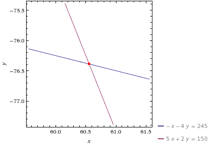### Example Question #1 : How To Find The Equation Of A Line

Solve the equation for x and y.

y + 5x = 40

x – y = –10

x = 12

y = 7

x = 15

y = 5

x = 5

y = 15

x = 7

y = 12

x = 5

y = 15

Explanation:

This one is a basic problem with two unknowns in two equations. Derive y=x+10 from the second equation and replace the y in first equation to solve the problem. So, x+10+5x=40 and x = 5. X-y= -10 so y=15. The graph below illustrates the solution.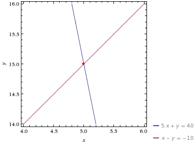### Example Question #1 : How To Find The Equation Of A Line

Solve the equation for x and y.

xy=30

x – y = –1

x = 4, 5

= –4, –5

x = –7, 3

y = 7, –3

x = 2, 3

y = 4, 5

x = 5, –6

y = 6, –5

x = 5, –6

y = 6, –5

Explanation:

Again the same process is required. This problem however involved multiplying x by y so is a bit different. We end up with two possible solutions. Derive y=x+1 and solve in the same manner as the ones above. The graph below illustrates the solution.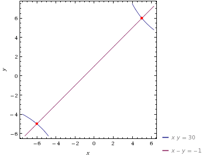### Example Question #11 : Slope And Line Equations

Solve the equation for x and y.

x/= 30

= 5

x = 150/31

y = 5/31

x = 5/150

y = 150/31

x = 2, 6

y = 3, 7

x = 7

y = 14

x = 150/31

y = 5/31

Explanation:

Similar problem to the one before, with x being divided by y instead of multiplied. Solve in the same manner but keep in mind the way that x/y is graphed. We end up solving for one solution. The graph below illustrates the solution,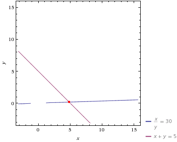### Example Question #131 : Coordinate Geometry

Solve the equation for x and y.

x – y = 26/17

2x + 3y = 2

x = 112/85

y = –18/85

x = 85/112

y = –85/18

x = 3

y = 2

x = –18/85

y = 112/85

x = 112/85

y = –18/85

Explanation:

Straightforward problem that presents two unknowns with two equations. The student will need to deal with the fractions correctly to get this one right. Other than the fraction the problem is solved in the exact same manner as the rest in this set. The graph below illustrates the solution.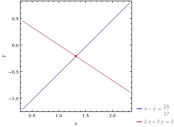### Example Question #11 : Psat Mathematics

What is the equation for a line with endpoints (-1, 4) and (2, -5)?

y = -x - 3

y = x + 3

y = 3x - 1

y = -3x + 1

y = -3x + 1

Explanation:

First we need to find the slope. Slope (m) = (y2 - y1)/(x2 - x1). Substituting in our values (-5 - 4)/(2 - (-1)) = -9/3 = -3 so slope = -3. The formula for a line is y = mx +b. We know m = -3 so now we can pick one of the two points, substitute in the values for x and y, and find b. 4 = (-3)(-1) + b so b = 1. Our formula is thus y = -3x + 1

### Example Question #1 : How To Find The Equation Of A Line

What is the equation of a line that goes through (4, 1) and (–2, –2)?

y = 2x – 1/2

y = x + 4

y = 4x – 1/2

y = 4x – 2

y = 1/2x – 1

y = 1/2x – 1

Explanation:

We will find the equation using slope intercept form: y=mx+b

1. Use the two points to find the slope.

The equation to find the slope of this line using two points is: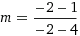Therefore, m = 1/2, so the slope of this line is 1/2.

2. Now that we have the slope, we can use one of the points that were given to find the y intercept. In order to do this, substitute y for the y value of the point, and substitute x for the x value of the point.

Using the point (–2, –2), we now have: –2 = (1/2)(–2) + b.

Simplify the equation to solve for b. b = –1

3. In this line m = 1/2 and b = –1

4. Therefore, y = 1/2x –1

### Example Question #1 : How To Find The Equation Of A Line

What line goes through the points (1, 1) and (–2, 3)?

2x – 4y = 6

3x + 5y = 2

3x + 2y = 6

2x + 3y = 5

3x – 2y = 5

2x + 3y = 5

Explanation:

Let P1 (1, 1) and P2 (–2, 3).

First, find the slope using m = rise ÷ run = (y2 – y1)/(x2 – x1) giving m = –2/3.

Second, substutite the slope and a point into the slope-intercept equation y = mx + b and solve for b giving b = 5/3.

Third, convert the slope-intercept form into the standard form giving 2x + 3y = 5.

### Example Question #2 : How To Find The Equation Of A Line

If angle A is 1/3 the size of angle B, then what is angle A?

45

43.1

22.5

30

27.4

22.5

Explanation:

From the image we can tell that angle a and angle b are complimentary

a + b = 90    and    3a = b

a + 3a = 90

a = 22.5

### Example Question #1 : How To Find The Equation Of A Line

What is the equation of the line with a negative slope that passes through the y-intercept and one x-intercept of the graph y = –x2 – 2x + 8 ?

y = –4x + 8

y = –4x + 4

y = –2x + 8

y = –2x + 4

y = –x + 8

y = –4x + 8

Explanation:

In order to find the equation of the line, we need to find two points on the line. We are told that the line passes through the y-intercept and one x-intercept of y = –x2 – 2x + 8.

First, let's find the y-intercept, which occurs where x = 0. We can substitute x = 0 into our equation for y.

y = –(0)2 – 2(0) + 8 = 8

The y-intercept occurs at (0,8).

To determine the x-intercepts, we can set y = 0 and solve for x.

0 = –x2 – 2x + 8

–x2 – 2x + 8 = 0

Multiply both sides by –1 to minimize the number of negative coefficients.

x+ 2x – 8 = 0

We can factor this by thinking of two numbers that multiply to give us –8 and add to give us 2. Those numbers are 4 and –2.

x+ 2x – 8= (x + 4)(x – 2) = 0

Set each factor equal to zero.

x + 4 = 0

Subtract 4.

x = –4

Now set x – 2 = 0. Add 2 to both sides.

x = 2

The x-intercepts are (–4,0) and (2,0).

However, we don't know which x-intercept the line passes through. But, we are told that the line has a negative slope. This means it must pass through (2,0).

The line passes through (0,8) and (2,0).

We can use slope-intercept form to write the equation of the line. According to slope-intercept form, y = mx + b, where m is the slope, and b is the y-intercept. We already know that b = 8, since the y-intercept is at (0,8). Now, all we need is the slope, which we can find by using the following formula: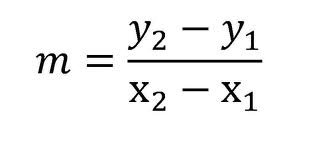m = (0 – 8)/(2 – 0) = –8/2 = –4

y = mx + b = –4x + 8

The answer is y = –4x + 8.

← Previous 1

### All PSAT Math Resources